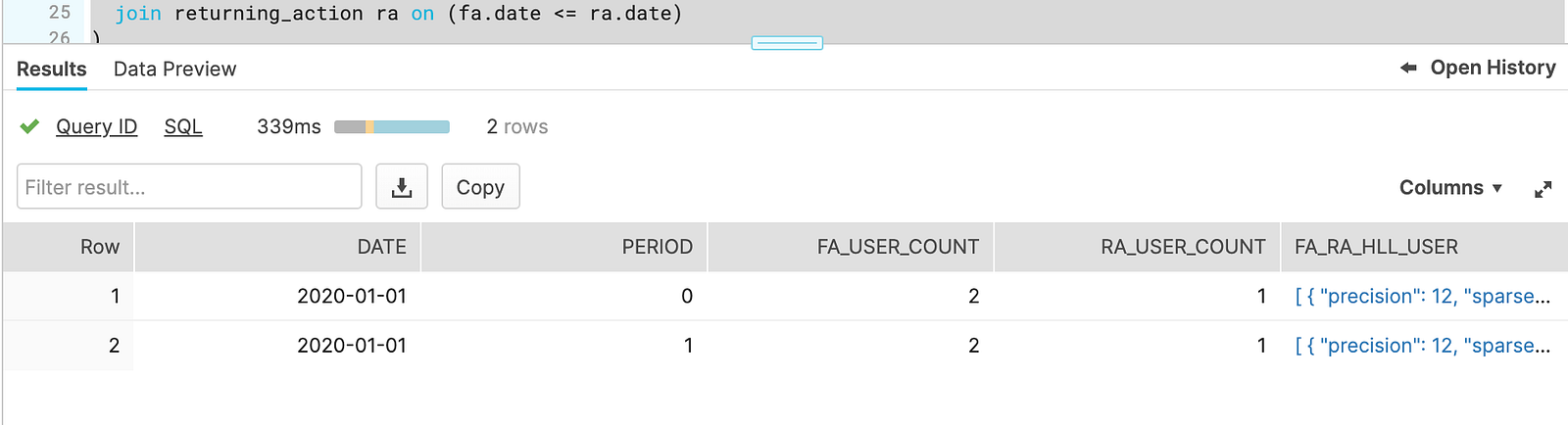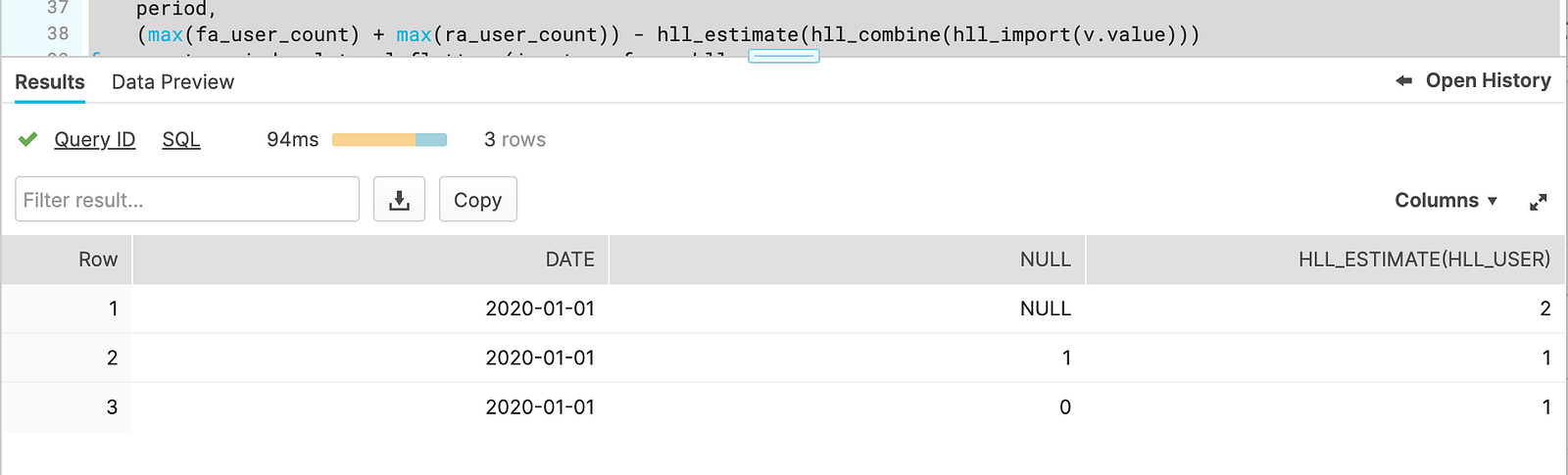# Probabilistic Retention Cohort SQL on Snowflake

TL;DR: Retention cohorts are expensive to generate, especially if you’re working on a database lacking analytic functions. A deterministic approach is; joining ‘first action’ to ‘returning action’ steps and counting the distinct connectors (user or device identifiers). Joining huge amounts of data should be avoided when working on big data. A better solution is to estimate the intersection of steps using the HyperLogLog algorithm, lets practice this on Snowflake’s HLL functions.

Snowflake is a cloud warehouse where you forget about maintenance, and focus on your query. They have a variety of analytic functions, a great console, programmable interfaces (npm package, JDBC driver). Loading data into Snowflake is easy for one time (COPY command) or continuously (Snowpipe). Truncated some table accidentally? They do time-travel ⌛️

### 1. The MinHash Approach

The similarity of the two sets can be calculated using the Jaccard Index. It is formulated as;

J(A,B) = |A ∩ B| / |A∪ B|

Although calculating the union and intersection can be an expensive operation over millions of rows. Minhash is an efficient solution that inputs your data into K distinct hash functions. For example, you choose K=1000 for your user identifier column with one million rows. Minhash will describe that set with 1000 dim.

A similarity index might sound a solution for plotting a retention cohort. Let’s see why we can’t use this solution, the similarity indexes on given cases are as follows;

• If A and B are the same = 1.
• If A and B are completely different = 0
• If A is a superset of B and 2.|A| = |B| = 0.5

To Exemplify the last case; in typical retention, we have the first step as `first_install` and `session_start` as the returning step. Which would be a vanilla retention query that you can find most of the platforms dealing with user event data. Session start event is the superset of first install events. Every user must install the app to start the session. Even though all the users are still there on day 0 for the session start, the Jaccard Index is not a solution that considers the overlaps. For more information and an example about MinHash on Snowflake checkout here.

### 2. Cardinality Estimation

The size of a set of elements is defined as cardinality in mathematics. In SQL we simply use the `count(distinct row)` function to calculate the number of distinctive rows. Imagine we’re filtering the set over some conditions, or even joining rows from another table. We might do the filtering on a column that is not clustered/partitioned, and your queries will start getting greedy on resources. The simplest solution in Snowflake is to replace `count distinct` to `approx_count_distinct` , which uses HyperLogLog to estimate distinct cardinality. Let’s consider the following query;

``````select sum(user_count) from (
select approx_count_distinct(column1) as user_count
from values
(1, DATE '2020-01-01'),
(1, DATE '2020-01-01'),
(2, DATE '2020-01-01'),

(2, DATE '2020-01-02')
group by date_trunc('day', column2)
)
// outputs: 3
``````

Different sets grouped by each day (maybe a case of materialization). For a reason, you need to aggregate the total unique users via summing. Sorry, the sum you seek is randomized by a factor of how frequently duplicates appear in your inner queries group by statement. There must be a way to group by your set and aggregate the correct cardinality, right? Yes!, by breaking down `approx_count_distinct` and using a combination of HyperLogLog functions that Snowflake provides for us.

``````select hll_estimate(hll_combine(user_count)) from (
select hll_accumulate(column1) as user_count
from values
(1, DATE '2020-01-01'),
(1, DATE '2020-01-01'),
(2, DATE '2020-01-01'),

(2, DATE '2020-01-02')
group by date_trunc('day', column2)
)
// outputs: 2
``````

The example above is very useful if you’re materializing cardinalities and aggregating later on. Also, check out HLL_EXPORT and HLL_IMPORT helpers to dump and import the binary representation into object format.

### 3. Composing Retention Query using HLL Functions

What is the number of users came back the next day? Assuming A is the first action and B the returning; Mathematically, the number of retained users can be described as the cardinality of intersection of A and B (|A⋂B|). Using HLL_COMBINE we can calculate the cardinality of unions, and substitute the intersections with;

``````|A⋂B| = |A| + |B| - |A⋃B|
``````

Let’s start with defining ‘first action’, ‘returning action’ and joining returning action day-by-day.

``````with first_action as (
select date_trunc('day', column2) as date,
hll_accumulate(column1) as hll_user
from values
(1, DATE '2020-01-01'),
(1, DATE '2020-01-01'),
(2, DATE '2020-01-01')
group by 1
),
returning_action as (
select date_trunc('day', column2) as date,
hll_accumulate(column1) as hll_user
from values
(1, DATE '2020-01-01'),
(1, DATE '2020-01-02')
group by 1
),
next_periods as (
select fa.date as date,
datediff('day', fa.date, ra.date) as period,
hll_estimate(fa.hll_user) as fa_user_count,
hll_estimate(ra.hll_user) as ra_user_count,
array_construct(hll_export(fa.hll_user), hll_export(ra.hll_user)) as fa_ra_hll_user
from first_action fa
join returning_action ra on (fa.date <= ra.date)
)
``````

The `next_periods` set joins the returning action by the date and calculates;

• The first appear date.
• The next period (in days, or weeks depending on how you truncated).
• Approximate count of first and returning action (via `hll_estimate(fa/ra.hll_user)`). To calculate intersection cardinality we need to subtract set cardinalities from the union cardinality.
• Aggregates first and returning actions HyperLogLog estimation, we’ll need those later on when using `hll_merge` to calculate union of A and B.select * from next_periods to the structure of the set.

To calculate the final form of the cohort, we will calculate the number of users for the same day (simply the first action) and union with merged and estimated HLL values of `next_periods` set.

``````select
date,
null,
hll_estimate(hll_user)
from first_action

union all

select
date,
period,
(max(fa_user_count) + max(ra_user_count)) - hll_estimate(hll_combine(hll_import(v.value)))
from next_periods, lateral flatten (input => fa_ra_hll_user) v
group by 1, 2
``````

Since we’re sure that date and period pairs are unique. Taking a maximum of `ra_user_count` and `fa_user_count` is okay. `hll_combine` is an aggregation function thus we either take the maximum of those counts or do this inside a subquery. Since we’ve only one day in the first action, the query result will show retention over the same and next day only.Query result for the retention cohort